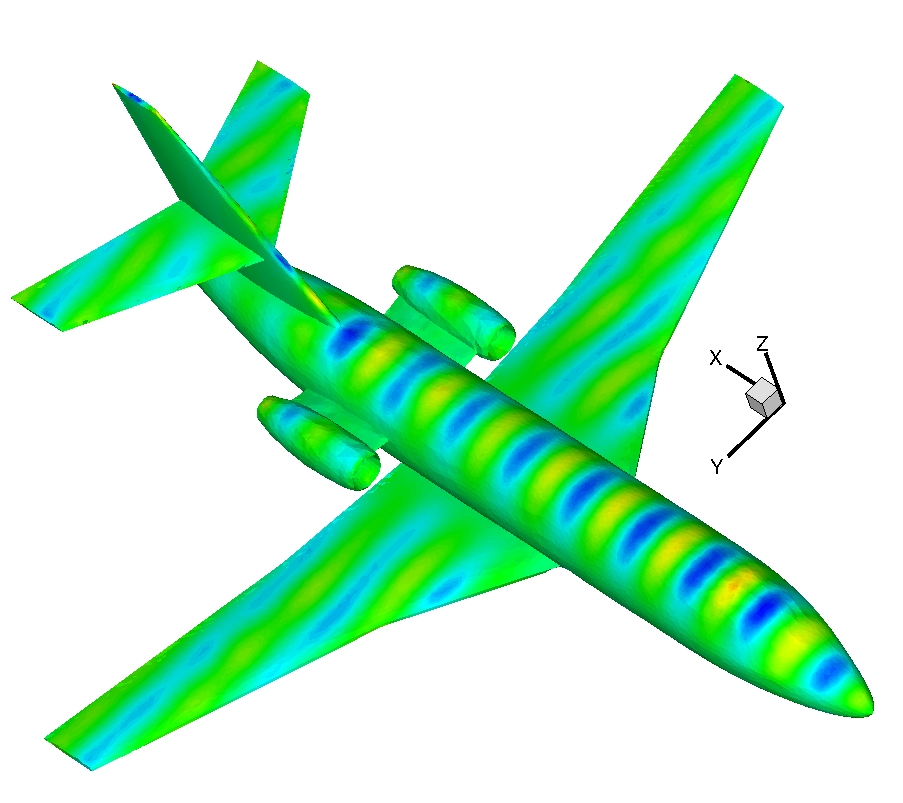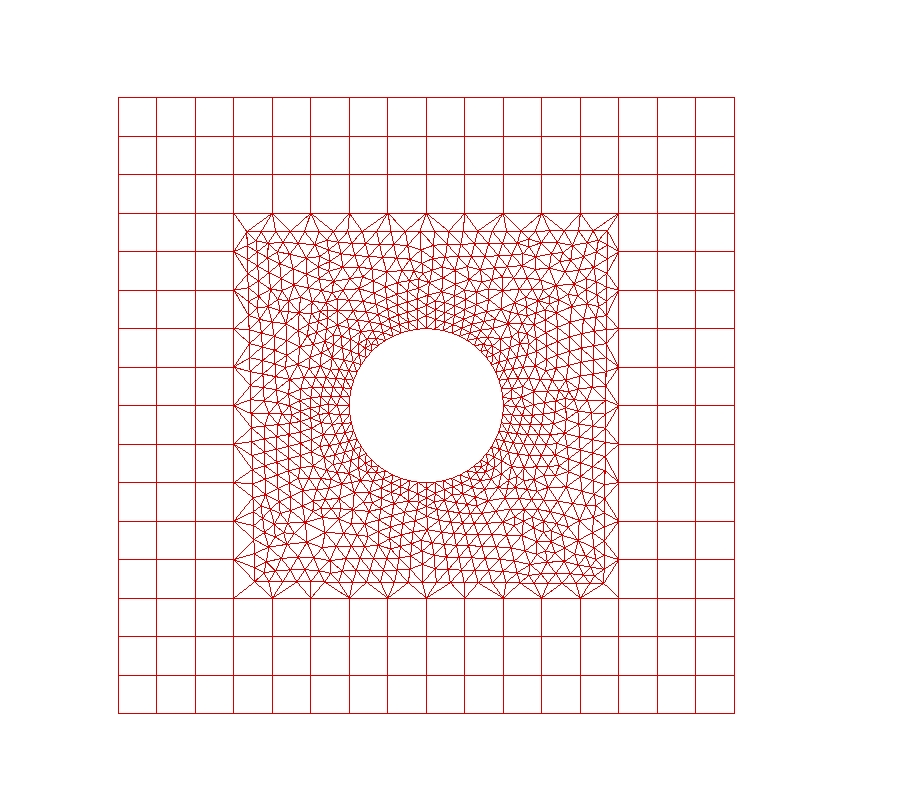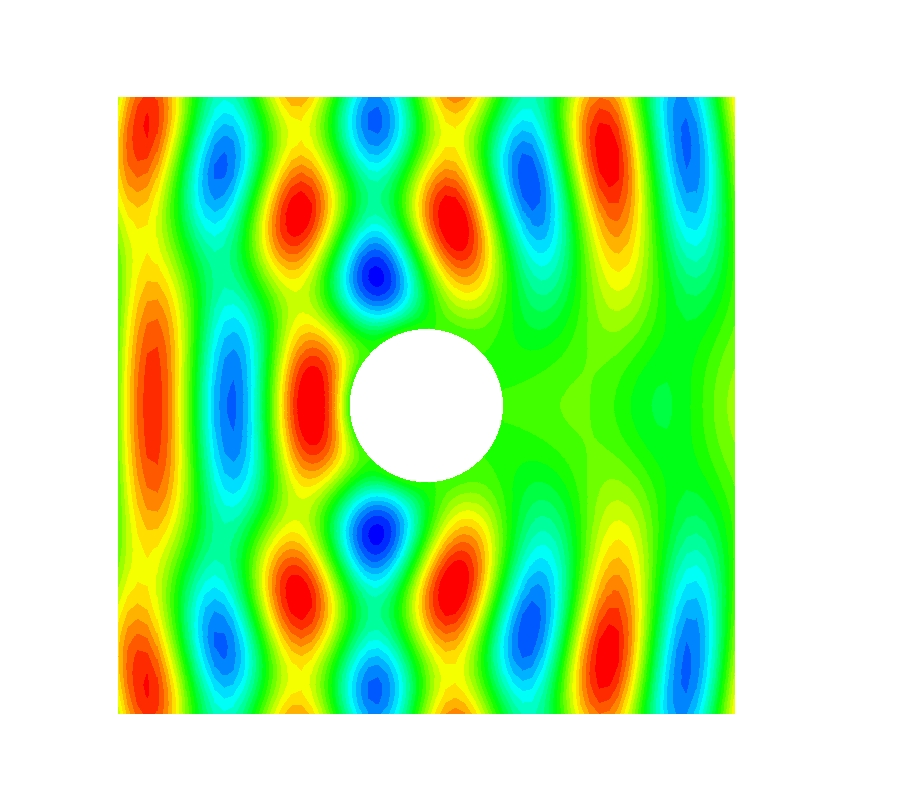EN FR
EN FR

## Section: New Results

### Discontinuous Galerkin methods for Maxwell's equations

#### DGTD-${ℙ}_{p}$ method based on hierarchical polynomial interpolation

Participants : Loula Fezoui, Joseph Charles, Stéphane Lanteri.

The DGTD (Discontinuous Galerkin Time Domain) method originally proposed by the team for the solution of the time domain Maxwell's equations  relies on an arbitrary high order polynomial interpolation of the component of the electromagnetic field, and its computer implementation makes use of nodal (Lagrange) basis expansions on simplicial elements. The resulting method is often denoted by DGTD-${ℙ}_{p}$ where $p$ refers to the interpolation degree that can be defined locally i.e. at the element level. In view of the design of a $hp$-adaptive DGTD method, i.e. a solution strategy allowing an automatic adaptation of the interpolation degree $p$ and the discretization step $h$, we now investigate alternative polynomial interpolation and in particular those which lead to hierarchical or/and orthogonal basis expansions. Such basis expansions on simplicial elements have been extensively studied in the context of continuous finite element formulations (e.g.  ) and have thus been designed with global conformity requirements (i.e. ${H}_{1}$, $H\left(\text{rot}\right)$ or $\left(\text{div}\right)$) whose role in the context of a discontinuous Galerkin formulation has to be clarified. This represents one of the objectives of this study.

Figure 3. Scattering of a plane wave by a business aircraft geometry computed by DGTD-${ℙ}_{1}$ method on a tetrahefdral mesh. Contour lines of the amplitude of the electric field.#### DGTD-${ℙ}_{p}{ℚ}_{k}$ method on multi-element meshes

Participants : Clément Durochat, Stéphane Lanteri, Claire Scheid, Mark Loriot [Distene, Pôle Teratec, Bruyères-le-Chatel] .

In this work, we study a multi-element DGTD method formulated on a hybrid mesh which combines a structured (orthogonal) quadrangulation of the regular zones of the computational domain with an unstructured triangulation for the discretization of the irregularly shaped objects. The general objective is to enhance the flexibility and the efficiency of DGTD methods for large-scale time domain electromagnetic wave propagation problems with regards to the discretization process of complex propagation scenes. As a first step, we have designed and analyzed a DGTD-${ℙ}_{p}{ℚ}_{k}$ method formulated on conforming hybrid quadrangular/triangular meshes for the solution of the 2D Maxwell's equations.

Figure 4. Scattering of a plane wave by a perfectly conducting cylinder computed by a DGTD-${ℙ}_{2}{ℚ}_{4}$ method on a hybrid triangular-quadrangular mesh.#### DGTD-${ℙ}_{p}$ method for dispersive materials

Participants : Claire Scheid, Maciej Klemm [Communication Systems & Networks Laboratory, Centre for Communications Research, University of Bristol, UK] , Stéphane Lanteri.

This work is undertaken in the context of a collaboration with the Communication Systems & Networks Laboratory, Centre for Communications Research, University of Bristol (UK). This laboratory is studying imaging modalities based on microwaves with applications to dynamic imaging of the brain activity (Dynamic Microwave Imaging) on one hand, and to cancerology (imaging of breast tumors) on the other hand. The design of imaging systems for these applications is extensively based on computer simulation, in particular to assess the performances of the antenna arrays which are at the heart of these systems. In practice, one has to model the propagation of electromagnetic waves emitted from complex sources and which propagate and interact with biological tissues. In relation with these issues, we study the extension of the DGTD-${ℙ}_{p}$ method originally proposed in  to the numerical treatment of electromagnetic wave propagation in dispersive media. We consider an approach based on an auxiliary differential equation modeling the time evolution of the electric polarization for a dispersive medium of Debye type (other dispersive media will be considered subsequently). This work comprises both theoretical aspects (stability and convergence analysis) of the resulting DGTD-${ℙ}_{p}$ method for the time domain Maxwell equations for dispersive media, and application aspects  .

#### DGFD-${ℙ}_{p}$ method for the frequency domain Maxwell equations

Participants : Victorita Dolean, Mohamed El Bouajaji, Stéphane Lanteri, Ronan Perrussel [Laplace Laboratory, INP/ENSEEIHT/UPS, Toulouse] .

For certain types of problems, a time harmonic evolution can be assumed leading to the formulation of the frequency domain Maxwell equations, and solving these equations may be more efficient than considering the time domain variant. We are studying a high order Discontinuous Galerkin Frequency Domain (DGFD-${ℙ}_{p}$) method formulated on triangular meshes for solving the 2D time harmonic Maxwell equations  . This work is undertaken in the context of the ANR MAXWELL project whose objective is the development of an ultra wideband georadar system for imaging the subsurface. In this context, the DGFD-${ℙ}_{p}$ method that we have proposed is used as the forward solver in an inversion process for the electric permittivity  .

#### Hybridized DGFD-${ℙ}_{p}$ method

Participants : Stéphane Lanteri, Liang Li, Ronan Perrussel [Laplace Laboratory, INP/ENSEEIHT/UPS, Toulouse] .

One major drawback of DG methods is their intrinsic cost due to the very large number of globally coupled degrees of freedom as compared to classical high order conforming finite element methods. Different attempts have been made in the recent past to improve this situation and one promising strategy has been recently proposed by Cockburn et al.  in the form of so-called hybridizable DG formulations. The distinctive feature of these methods is that the only globally coupled degrees of freedom are those of an approximation of the solution defined only on the boundaries of the elements. This work is concerned with the study of such Hybridizable Discontinuous Galkerkin (HDG) methods for the solution of the system of Maxwell equations in the time domain when the time integration relies on an implicit scheme, or in the frequency domain. As a first setp, HDGTD and HDGFD  methods have been developed for the solution of the 2D propagation problems.

#### Exact transparent condition in a DGFD-${ℙ}_{p}$ method

Participants : Mohamed El Bouajaji, Nabil Gmati [ENIT-LAMSIN, Tunisia] , Stéphane Lanteri, Jamil Salhi [ENIT-LAMSIN, Tunisia] .

In the numerical treatment of propagation problems theoretically posed in unbounded domains, an artificial boundary is introduced on which an absorbing condition is imposed. For the frequency domain Maxwell equations, one generally use the Silver-Müller condition which is a first order approximation of the exact radiation condition. Then, the accuracy of the numerical treatment greatly depends on the position of the artificial boundary with regards to the scattering object. In this work, we have conducted a preliminary study aiming at improving this situation by using an exact transparent condition in place of the Silver-Müller condition. Promising results have been obtained in the 2D case and call for an extension of this work to the more challenging 3D case.